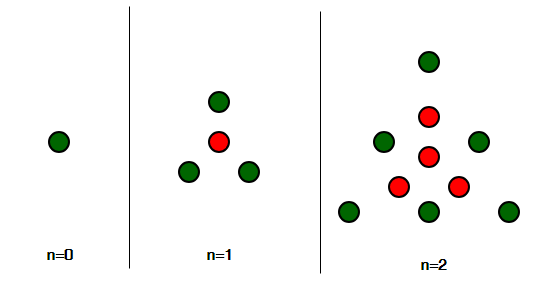Open in App
Not now

# Centered triangular number

• Difficulty Level : Basic
• Last Updated : 18 May, 2022

Given an integer n, find the nth Centered triangular number.
Centered Triangular Number is a centered polygonal number that represents a triangle with a dot in the centre and all other dots surrounding the centre in successive triangular layers [Source : Wiki ]

Pictorial Representation :The first few centered triangular number series are :
1, 4, 10, 19, 31, 46, 64, 85, 109, 136, 166, 199, 235, 274, 316, 361, 409, 460………………………..

Examples:

```Input : n = 1
Output : 4
Explanation :
A dot in the centre and 3 dots forming the
triangle outside it, thus 4.

Input : n = 6
Output : 64

Input : n = 10
Output : 166```

Approach
nth Term of centered triangular number is given by:

``

Basic Implementation of the above approach:

## C++

 `// C++ Program to find the` `// nth Centered Triangular number` `#include ` `using` `namespace` `std;`   `// function for Centered` `// Triangular number` `int` `Centered_Triangular_num(``int` `n)` `{` `    ``// formula for find Centered` `    ``// Triangular number nth term` `    ``return` `(3 * n * n + 3 * n + 2) / 2;` `}`   `// Driver Code` `int` `main()` `{` `    ``// For 3rd Centered Triangular number` `    ``int` `n = 3;` `    ``cout << Centered_Triangular_num(n) << endl;`   `    ``// For 12th Centered Triangular number` `    ``n = 12;` `    ``cout << Centered_Triangular_num(n) << endl;`   `    ``return` `0;` `}`

## C

 `// C Program to find the` `// nth Centered Triangular number` `#include `   `// function for Centered` `// Triangular number` `int` `Centered_Triangular_num(``int` `n)` `{` `    ``// formula for find Centered` `    ``// Triangular number nth term` `    ``return` `(3 * n * n + 3 * n + 2) / 2;` `}`   `// Driver Code` `int` `main()` `{` `    ``// For 3rd Centered Triangular number` `    ``int` `n = 3;` `    ``printf``(``"%d\n"``,Centered_Triangular_num(n));`   `    ``// For 12th Centered Triangular number` `    ``n = 12;` `    ``printf``(``"%d\n"``,Centered_Triangular_num(n));`   `    ``return` `0;` `}`   `// This code is contributed by kothavvsaakash.`

## Java

 `// Java Program to find ` `// the nth Centered ` `// Triangular number` `import` `java.io.*;`   `class` `GFG ` `{` `    `  `// function for Centered` `// Triangular number` `static` `int` `Centered_Triangular_num(``int` `n)` `{` `    ``// formula for find Centered` `    ``// Triangular number nth term` `    ``return` `(``3` `* n * n + ` `            ``3` `* n + ``2``) / ``2``;` `}`   `// Driver Code` `public` `static` `void` `main (String[] args) ` `{`   `// For 3rd Centered ` `// Triangular number` `int` `n = ``3``;` `System.out.println(Centered_Triangular_num(n));`   `// For 12th Centered ` `// Triangular number` `n = ``12``;` `System.out.println(Centered_Triangular_num(n));` `}` `}`   `// This code is contributed by ajit`

## Python3

 `# Program to find nth ` `# Centered Triangular number`   `def` `Centered_Triangular_num(n) :` `    `  `    ``# Formula to calculate nth` `    ``# Centered Triangular number` `    ``return` `(``3` `*` `n ``*` `n ``+` `            ``3` `*` `n ``+` `2``) ``/``/` `2`   `# Driver Code` `if` `__name__ ``=``=` `'__main__'` `:`   `    ``# For 3rd Centered ` `    ``# Triangular number     ` `    ``n ``=` `3` `    ``print``(Centered_Triangular_num(n))` `    `  `    ``# For 12th Centered` `    ``# Triangular number` `    ``n ``=` `12` `    ``print``(Centered_Triangular_num(n))` `                `  `                `  `# This code is contributed ` `# by akt_mit`

## C#

 `// C# Program to find ` `// the nth Centered ` `// Triangular number` `using` `System;`   `class` `GFG` `{`   `// function for Centered` `// Triangular number` `static` `int` `Centered_Triangular_num(``int` `n)` `{` `    ``// formula for find Centered` `    ``// Triangular number nth term` `    ``return` `(3 * n * n + ` `            ``3 * n + 2) / 2;` `}`   `// Driver Code` `static` `public` `void` `Main ()` `{`   `// For 3rd Centered ` `// Triangular number` `int` `n = 3;` `Console.WriteLine(Centered_Triangular_num(n));`   `// For 12th Centered ` `// Triangular number` `n = 12;` `Console.WriteLine(Centered_Triangular_num(n));` `}` `}`   `// This code is contributed by akt_mit`

## PHP

 ``

## Javascript

 ``

Output :

```19
235```

Time Complexity: O(1)
Auxiliary Space: O(1)

My Personal Notes arrow_drop_up
Related Articles